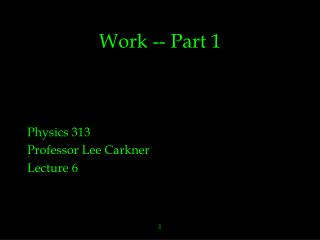DownloadDownload PresentationWork -- Part 1

# Work -- Part 1

Download Presentation## Work -- Part 1

- - - - - - - - - - - - - - - - - - - - - - - - - - - E N D - - - - - - - - - - - - - - - - - - - - - - - - - - -
##### Presentation Transcript

1. Work -- Part 1 Physics 313 Professor Lee Carkner Lecture 6 1

2. Work • Work is force times displacement • In thermodynamics we will only consider external work • Involves interaction with another system or its surroundings (external to the system) 2

3. Sign Conventions • Work by the system is negative • decrease internal energy • Work done on the system is positive • increase internal energy • If one system does work on the other, the sign depends on point of view 3

4. Work and Hydrostatic Systems • Work is not a property of the system • Neither is heat • P, V and T are • Work is a transfer of energy due to a volume change • Heat is a transfer of energy due to temperature change 4

5. Work, Pressure and Volume W = F x dW = F dx dW = PA dx dW = -P dV • A small volume change (dV) produces a small amount of work (dW) • If dV is positive (increase in V) then W is negative (work by the system) 5

6. Total Work • To find the total work, integrate dW between the initial and final states: W = - P dV • Need to know P as a function of V • Equation of state • Need to limit T • W depends on both the change of volume and how the volume changed 6

7. PV Diagram • For a change of volume, (Vi, Pi) and (Vf,Pf) can be plotted on a PV diagram • The process by which the volume changes is a line or curve connecting the two points • The work is the area under the curve • For different processes, different curves, different amounts of work 7

8. Closed Cycle • If the system moves from i to f and then back to i, it is a cycle • If the same path is traveled in both directions, W=0 • if two different paths are traveled W is the area between the curves 8

9. Path Dependence • What are the paths? • Isothermal: keep constant T (add or subtract heat) • follow isotherm • Isobaric: constant P (add or subtract heat) • horizontal • Isochoric: keep constant volume (rigid container, W=0) • vertical 9

10. P-V Diagram Isobaric (p=const.) p Isothermal (T=const) Adiabatic (Q=0) Isochoric (V=const) V 10

11. Sign of Work • On PV diagram: • Move to right = compression = positive work • Move to left = expansion = negative work 11

12. Calculating Work • Need equation of state and limit on T • Example: isothermal • P is replaced with equation of state, T comes out of integral • Final expression for work in terms of constants and Vi and Vf 12

13. Ideal Gas: Isothermal Process PV = nRT P = nRT/V W = - (nRT/V) dV W = -nRT  (1/V) dV W = -nRT ln (Vf/Vi) 13

14. Procedures • From equations: • Find P(V,T) • Limit T • Integrate dW • Check sign • From PV diagram: • Find area • Find sign (V increase (-) or decrease(+)) 14